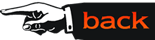## Geometric Structures

Preservice teachers are especially motivated when the mathematics covered in a course is directly related in meaningful ways to materials that they expect to someday cover as mathematics teachers. It is crucial that future middle grade mathematics teachers have a deep understanding of Euclidean geometry and how this geometry relates to the real world.

All of the NSF supported standards-based projects contain rich and engaging geometry units. One good approach in introducing standards-based curriculum materials to a preservice geometry class is to have a team of 4 to 5 students present part of a unit from one of the curricula to the rest of the class. For example, students could choose a part of a unit from Getting in Shape (MathScape), having to do with symmetries, or part of a unit from Triangles and Patchwork (Mathematics in Context) dealing with similarity. Each of these units cover topics which will be studied in the college class on a deeper level. An essential part of the team presentation is to explain the fundamental mathematical ideas behind the material they present. This demonstrates the real role that geometry plays in these standards-based materials and also impresses on future teachers that it is very important to make sure middle school students learn the mathematics behind the activities and investigations.

The Connected Mathematics Project has a unit entitled Stretching and Shrinking which studies similarity by using a number of activities that can be accomplished in a middle school classroom. For example, in Investigation 2 of Stretching and Shrinking middle school students examine similarities in the xy-plane using graph paper. Given one figure defined using coordinates for corners of the figure, middle school students use simple rules to construct related figures and then decide which new figures are similar to the original figure and which are not. For example, one new figure comes from defining the transformation (x,y) goes to (2x,2y) and another new figure comes from the transformation (x,y) goes to (3x,y). When future middle school teachers thoroughly explore and scrutinize this investigation, they quickly come to understand the need for middle school mathematics teachers to have a strong mathematical background in coordinate geometry and transformations. It also provides a natural opportunity to engage in proportional reasoning, an important cognitive process that is challenging for middle school teachers. This similarity investigation can be covered early in the semester and it then establishes a foundation for the study of groups of (geometric) transformations encountered later in the course.

Similarity can also be studied later in the course when the Geometers Sketchpad (GSP) is introduced. The GSP is very user friendly and is particularly useful in studying similar figures and similarity transformations. The prospective middle grade teachers readily grasp how easy it is it to use the GSP as an effective aid for learning standards-based materials. It is instructive in the college geometry classroom to have a team of 4 or 5 preservice teachers present a lesson to the class illustrating how one might integrate the use of the GSP in teaching middle school students a unit on some aspect of geometry such as similarity.

A further mathematical reason justifying a revisit of similarity is the study of spherical geometry. Although similarity is of great importance in Euclidean geometry, it is equivalent to congruence in spherical geometry. Since there are no similarity transformations which have proportionality factor other than one for this geometry, the only similarity transformations in spherical geometry are isometries. In spherical geometry, it is easy to illustrate the failure of the usual SAS similarity criterion that Euclidean geometry enjoys. In particular, given two spherical triangles with two sides in the same proportion (with a constant of proportionality different from one) and the include angles congruent, then the triangles are not similar. In fact, since in spherical geometry the excess angle sum of a triangle is directly proportional to its area, it is clear that the usual proportional side and included angle similarity criterion can hold only when the proportionality factor is exactly one. The prospective middle school teachers gain a much better idea of the underlying structure of Euclidean geometry by comparing results, such as in this spherical example, which are fundamentally different from the standard Euclidean conclusions.